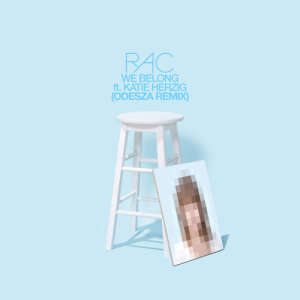# We Belong

9 Feb 2015

## We Belong Lyrics

We Belong (Odesza Remix) - RAC/Katie Herzig (凯蒂·赫奇格)

There's a way that you look me straight in the eyes

Only last for a moment it last through the night

It's so easy to hold you

You're so easy to mold to

It's a dream to be close to you

Didn't know you were meant for me

Be until you came to me

Until it came to be

Until I came to see

Until you came to be

We belong to where we're going

Where we're going

We belong to where we're going

Where we're going

We belong to where we're going

Where we're going

We belong to where we're going

Where we're going

We belong to where we're going

Where we're going

We belong to

Where we're going where we're going

We belong to

Where we're going where we're going

We belong to

Where we're going where we're going

We belong to